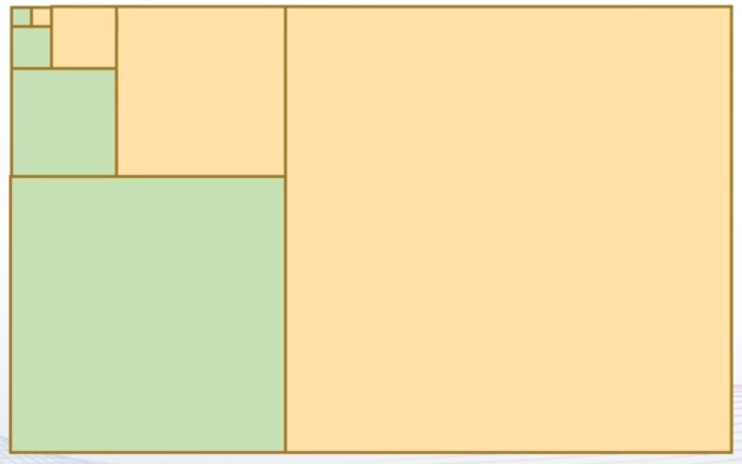• MATLAB递归函数

万次阅读 多人点赞 2019-04-05 12:17:38
MATLAB递归函数 递归函数就是函数调用自己本身，具体来说就是在一个函数模块里（设函数模块为factor.m，），存在函数（factor），这个函数（factor）用来调用函数模块（factor.m）。看下面的例子： 递归函数求n的...

MATLAB递归函数

递归函数就是函数调用自己本身，具体来说就是在一个函数模块里（设函数模块为factor.m，），存在函数（factor），这个函数（factor）用来调用函数模块（factor.m）。看下面的例子：

递归函数求n的阶层
创建函数文件factor.m

function f=factor(n)       % function 输出形参表 = 函数名（输入形参表）；
if n<=1                          % 停止递归函数调用条件
f=1;
else
f=n*factor(n-1)；
end
在这里插入代码片

设输入n=5时，即在交互式命令窗口输入
factor(5)
ans =

120

具体递归函数调用过程可以这么理解：
当输入n=5时；

f=5factor(4) ; factor（4）调用函数模块factor.m；即相当于n=4,则有：
f=factor(4)=4
factor(3) ; 同理，factor（3）调用函数模块factor.m，则有：
f=factor(3)=3factor(2) ;
f=factor(2)= 2
factor(1) ; factor(1)调用函数模块factor.m，因为 n<=1, 故得 f=1返回给f=factor(1),即f=factor（1）=1;接下来factor（1）的值返回给factor（2），一次类推，factor（2）返回给factor（3）…

将上面代码整理一下：

f=5factor(4) ; (1)
factor(4)=4
factor(3) ; (2)
factor(3)=3factor(2) ; (3)
factor(2)= 2
factor(1) ; (4)
factor(1)=1; (5)

将过程(5)合并到（4），（4）合并到（3）.........最终合并到（1）得：f=5*4*3*2*1=120

即调用过程：从(1)→(2)→(3)→(4)→(5)→(4)→(3)→(2)→(1)
为了更好的理解这个过程，我们将程序每一次的计算结果输出：
MATLAB函数文件factor.m：
function f=factor(n)
if n<=1
f=1;
else
f=n*factor(n-1);
end
f

在命令窗口输入：factor(5)
运行结果是：
f =
1
f =
2
f =
6
f =
24
f =
120
ans =
120

结论：就是递归函数调用一层一层剥开他的衣服，剥光见到皮皮虾纹身以后又让他按照剥开衣服的你顺序一层一层的穿回去。

展开全文• 在正整数集定义如下迭代序列 n=n/2 若n为偶数；n=3n+1 若n为奇数 从小于一百万的数开始，能够生成最长序列的是哪个数？ 例如：13-40-20-10-5-16-8-4-2-1（10次） 文件包含迭代思路递归迭代
• 我正在努力完成这项任务：创建一个递归函数,它将n作为参数并创建一个这样的矩阵,在这种情况下n = 3：0 1 2 3 2 1 01 1 2 3 2 1 12 2 2 3 2 2 23 3 3 3 3 3 3我...

我正在努力完成这项任务：

创建一个递归函数,它将n作为参数并创建一个这样的矩阵,在这种情况下n = 3：

0 1 2 3 2 1 0

1 1 2 3 2 1 1

2 2 2 3 2 2 2

3 3 3 3 3 3 3

我已经想出了这个：

function AA = A(n)

if n == 0

AA (1,1) = 0;

else

AA = n*ones(n+1,2*n+1);

AA(1:n, [1:n, n+3:end]) = A(n-1);

end

end

但输出似乎在RHS上有一个奇怪的转变：

0 1 2 3 3 2 1

1 1 2 3 3 2 1

2 2 2 3 3 2 2

3 3 3 3 3 3 3

有人可以帮忙吗？

展开全文• Matlab中递归函数调用详解

千次阅读 2021-04-18 09:51:13
利用单独的M文件并递归地调用该文件，既可以避免多个M文件的复杂性，又可以利用函数的优点。使用开关 switches或if elseif语句，可将回调函数装入调用函数内。通常这样一种函数调用的结构为function guifunc(switch)...

利用单独的M文件并递归地调用该文件，既可以避免多个M文件的复杂性，又可以利用函数的优点。使用开关 switches或if  elseif语句，可将回调函数装入调用函数内。

通常这样一种函数调用的结构为

function guifunc(switch)。

其中switch确定执行哪一个函数开关的参量，它可以是字符串 ' startup ' ， ' close ' ， ' sectolor ' 等等，也可以是代码或数字。如switch是字符串，则可如下面所示的M文件片段那样将开关编程。

if nargin < 1， switch =  ' startup ' ； end；

if ~isstr(switch)， error( ' Invalid argument ' )， end；

if strcmp(switch， ' startup ' )，

elseif strcmp(switch， ' setcolor ' )，

elseif strcmp(switch， ' close ' )，

end

如果是代码或字符串，开关也可以相同方式编程。

if nargin < 1， switch = 0； end；

if isstr(switch)， error( ' Invalid argument ' )， end；

if switch = = 0，

elseif switch = =1，

elseif switch ==2，

end

下面的例子说明了方位角滑标如何可作为单独的函数M文件来实现：

function setview(switch)

if nargin < 1， switch =  ' startup ' ； end；

if ~isstr(switch)， error( ' Invalid argument. ' )； end；

vw = get(gca， ' view ' )； % This information is needed in both sections

if strcmp(switch， ' startup ' ) % Define the controls and tag them

Hc_az = uicontrol(gcf， ' Style ' ， ' slider ' ，...

' Position ' ，[10 5 140 20]，...

' Min ' ，-90， ' Max ' ，90， ' Value ' vw(1)，...

' Tag ' ， ' AZslider ' ，...

' Callback ' ， ' setview( ' set ' ) ' )；

Hc_min=uicontrol(gcf， ' Style ' ， ' text ' ，...

' Position ' ，[10 25 40 20]，...

' String ' ，num2str(get(Hc_az， ' Min ' )))；

Hc_max = uicontrol(gcf， ' Style ' ， ' text ' ，...

' Position ' ，[110 25 40 20]，...

' String ' ，num2str(get(Hc_az， ' Max ' )))；

Hc_cur =uicontrol(gcf， ' Style ' ， ' text ' ，...

' Position ' ，[60 25 40 20]，...

' Tag ' ， ' AZcur ' ，...

' string ' ，num2str(get(Hc_az， ' Value ' )))；

elseif strcmp(switch， ' set ' ) % Execute the Callback

Hc_az=findobj(gcf， ' Tag ' ， ' AZslider ' )；

Hc_cur-findobj(gcf， ' Tag ' ， ' AZcur ' )；

str = num2str(get(Hc_az， ' Value ' ))；

newview - [get(Hc_az， ' Value ' ) vw(2)]；

set(Hc_cur， ' String ' ， str)

set(gca， ' View ' ，newview)

end

上述的两个例子均设置了 ' tag ' 属性，利用该属性和函数findobj寻找回调函数所需对象的句柄。

展开全文• 使用递归函数求一个数的阶乘。 复制 MATLAB 工作空间文件夹中的函数文件并用作因子（任意数字） 结果将显示在下面matlab
• matlab递归数列求和GUI,自编写，matlab递归数列求和GU,亲测可用matlab递归数列求和GUmatlab
• matlab函数的递归调用

千次阅读 2020-07-28 15:04:08
matlab函数递归调用 1、求n的阶乘 函数文件fact.m function f=fact (n) if n<=1 f=1; else f=fact (n-1)*n; end 脚本文件a.m n=input('Please input n='); s=fact (n); disp(s) 2、斐波拉契数列 ...

matlab函数的递归调用

1、求n的阶乘

函数文件fact.m
function f=fact (n)
if n<=1
f=1;
else
f=fact (n-1)*n;
end

脚本文件a.m
s=fact (n);
disp(s)

2、斐波拉契数列

Fibonacci数列定义如下：
f1=1
f2=1
fn=fn-1+fn-2   （n>2）
编写递归调用函数求Fibonacci数列的第n项，然后调用该函数验证Fibonacci数列的如下性质：
f1^2+f2^2+f3^2+……+fn^2=fn×fn+1

函数文件ffib.m
function f=ffib(n)
if n>2
f=ffib(n-1)+ffib(n-2);
else
f=1;
end

脚本文件test.m
F=[];
for k=1:20
F=[F,ffib(k)*ffib(k)]; %追加
end
sum(F)
ffib(20)*ffib(21)

注：该性质可用如下的图形解释，等式两边分别是面积的两种算法（小面积之和=总面积）。展开全文matlab 矩阵 算法
• 我知道这不是匿名函数的用途，但就像我试图通过匿名函数创建递归函数一样。 递归函数的原型显然是阶乘函数。 问题是在匿名函数中很难区分大小写。 到目前为止我设法做的是：f=@(cn,n,f)eval('if n>1; f(cn*n,n-1,...
• function temserch(t,yzd) global fhx bs tem u; %%%%%%%%%%%%%%%%% 寻找连接矩阵tem %%%%%%%%%%%%%%% jj=... end end end return 这个函数中我就重复调用了temserch函数，最后如果一级级返回的话可能会很麻烦啊！
• 本帖最后由 xiaobai1123q 于 2017-8-11 22:47 编辑我用matlab实现了一下simrank算法，使用稀疏矩阵保存节点间的引用关系，但是在100个节点的时候，就开始崩了，提示我说内存不足，可能存在死循环，值得一提的是虽然...
• 函数递归调用 一个函数调用它自身称为函数递归调用。 递归是把一个大型复杂的问题层层转化为一个与原问题相似的规模较小的问题来求解。大大地减少了程序的代码量，但递归调用也会加大存储空阃和执行时间的开销，...matlab
• 最小二乘滤波算法的基本算法是递归最小二乘算法，这种算法实际上是FIR维纳滤波器的一种时间递归实现，它是严格以最小二乘准则为依据的算法。它的主要优点是收敛速度快，所以在快速信道均衡、实时系统辨识和时间序列...最小二乘
• 函数 [varargout] = union_several (varargin) % 函数 [varargout] = union_several (varargin) % varargout{1} 是联合向量% varargout{i>1} 是各种 varargin 的索引（按它们的顺序） numarr = 长度（可变参数）； ...matlab
• 递归函数两大特点： 1.能够调用函数自身 2.至少有一个出口（结束函数自身调用） 函数实现： def calnum(num): if num != 1: # 递归调用自身函数 csum = num * calnum(num - 1) else: # 设置递归出口 csum = 1...
• 用于电子传输的递归格林函数方法的 100 行 MATLAB 代码 特征 这是一个简单但有效的代码，用于计算安德森方格模型中的电子传输。 此代码用于在评论文章（待提交）中进行一些计算。 档案组织 一个测试脚本： test_...
• function y = fib( n ) %UNTITLED2 Summary of this function goes here % Detailed explanation goes here if ( n == 1 || n== 2 )  y=1;  return ;   else y = fib(n -1) + fib( n -2 ) ;
• php递归函数中使用return的时候会碰到无法正确返回想要的值得情况，如果不明白其中的原因，很难找出错误的，就下面的具体例子来说明一下吧： 复制代码 代码如下: function test($i){$i-=4; if($i<3){ return$i;...
• 函数递归调用 定义：一个函数调用它自身成为函数递归调用。递归是一个大型的问题层层转化为一个与原问题相似的规模较小的问题来求解。 优点：大大减少代码量 缺点：加大存储空间和执行时间的开销 递归调用的两...
• matlab中分段函数代码PLRNNstsp 分段线性递归神经网络（PLRNN）模型中用于状态推断和参数估计的Matlab代码 该文件夹包含MatLab代码和来自 Durstewitz，D（2017）一种用于分段线性递归神经网络的状态空间方法，用于从...
• 函数文件的基本结构 function 输出形参表=函数名（输入形参表） 注释说明部分 函数体语句 注意：当多个形参时，形参之间用逗号分隔，组成形参表。当输出形参多于一个时，应该用方括号括起来，构成一个输出矩阵。 ...matlab
• y = walshGenerator(N,K) 此函数确定 2^K 阶离散沃尔什函数数 N 使用递归算法来降低内存需求。... 在科英布拉大学（葡萄牙）的单像素成像项目下开发的 Matlab 函数。 如果您在工作中使用此功能，请引用为：matlab
• matlab利用递归求解差分方程时间：2018-5-23matlab利用递归求解差分方程function y = recur(a,b,n,x,x0,y0);%% y = recur(a,b,n,x,x0,y0)% solves for y[n] from:% y[n] + a1*y[n-1] + a2*y[n-2]...+ an*y[n-N]% = ...
• Matlab代码无需使用任何内置函数即可递归查找数字的总和。matlab
• 这个函数从一个像素开始，如果它是 1，它会找到 4 个连接中的所有邻居并给它们相同的标签然后为每个调用本身大图像中的一些问题仅限于 500 的递归，所以我假设在 c++ 中实现它可能会导致一些堆栈溢出，一种解决方案...matlab
• Line Simplificationdpsimplify uses the recursive Douglas-Peucker line simplification algorithm to reduce the number of vertices in a polyline according to a specified tolerance. The algorithm is also ...
• matlab开发-递归目录函数执行。在执行所提供函数的所有目录中循环数学
• MATLAB一个递归实例

万次阅读 2016-10-26 11:02:11
MATLAB一个递归实例 昨天在MATLAB中文论坛上见到的一个小题目，很适合用递归来解决。 题目描述输入一个方阵，把所有的数按照逆时针方向旋转一个位子。 例如，将矩阵 ⎡⎣⎢147258369⎤⎦⎥ \left[ \begin{array}...matlab 递归
• matlab 递归深度问题mip版关注:259答案:2悬赏:20解决时间 2021-01-18 14:23已解决2021-01-17 18:00matlab 在并行操作中，对多个矩阵进行特征分解，出现了这个：Maximum recursion limit of 500 reached. Use set(0,'...
• 所以我写了这个函数递归调用MATLAB的多个输入的hypot函数： 用法：Y = HYPOTR(X) 或 Y = HYPOTR(X1, X2, X3, ...) 此函数与内置 MATLAB 函数 hypot 的工作方式相同，但允许通过递归进行两个以上的输入（或单个...matlab...

matlab递归函数代码matlab 订阅